# C++ program to convert uppercase to lowercase

This article will teach you how to convert uppercase characters to lowercase characters in C++ and provide you with code to do so. The program is created with and without using the standard library (or string) function. Here is the list of programs:

### Uppercase character

An uppercase character is basically an alphabet character written in capital letters. For example, the ASCII values of all 26 uppercase alphabet characters are 65–90. Where 65 is the ASCII value of A, 66 is the ASCII value of B, and so on up to 90, which is the ASCII value of Z.

### Lowercase character

A lowercase character is basically an alphabet character written in small letters. For example, the ASCII values of all 26 lowercase alphabet characters are 97–122. That is, a→97, b→98, c→99, and so on.

### Uppercase and lowercase string

If all the characters available in a string are in uppercase, then it is called an "uppercase string." And if all the characters of a string are written in lowercase, then it is called a lowercase string.

Note: A "string" is a combination of more than one character. For example, CODESCRACKER (an uppercase string) or codescracker (a lowercase string).

### Uppercase to lowercase Formula

since the ASCII value of A (a capital letter) is 65 and the ASCII value of a (a small letter) is 97. The difference between them is 32. Therefore, by adding 32 to the ASCII value of a capital letter, say A, we'll get the ASCII value of its equivalent letter in lowercase. That is,:

`65 (ASCII value of A) + 32 = 97 (ASCII value of a)`

## C++ Uppercase character to lowercase

To convert a character from uppercase to lowercase in C++ programming, you have to ask the user to enter a character in uppercase and then convert it into its equivalent lowercase character. Finally, print the equivalent character in lowercase on the output.

```#include<iostream>
using namespace std;
int main()
{
char chUpper, chLower;
int ascii;
cout<<"Enter the Character (in Uppercase): ";
cin>>chUpper;
ascii = chUpper;
ascii = ascii+32;
chLower = ascii;
cout<<"\nIts Lowercase: "<<chLower;
cout<<endl;
return 0;
}```

This program was built and runs under the Code::Blocks IDE. Here is its sample run:Now supply the character input in uppercase, say C, and press the ENTER key to print its equivalent character in lowercase as shown in the output given below: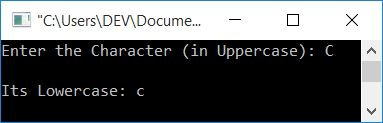In the above program, when the user enters C as an uppercase character input, then it gets stored in the chUpper variable. So the statement given below is:

`ascii = chUpper;`

Initializes the ASCII value of C to ascii. As a result, ascii = 67. Now the statement:

`ascii = ascii+32;`

initializes 67+32 or 99 to ascii. So the new value of ascii is 99, which is the ASCII value of c (lower case). So the statement is:

`chLower = ascii;`

Because chLower is a char (character) variable, it is initialized with the character that corresponds to the ASCII value of 99. So chLower=c. Now print the value of chLower as output.

### How to deal with an invalid input

If the user enters an invalid input, like an alphabet character that is already in lowercase or any other character like a number or a special character, then follow the program given below. The previous program does not work correctly in this case.

```#include<iostream>
using namespace std;
int main()
{
char chUpper, chLower;
int ascii;
cout<<"Enter the Character: ";
cin>>chUpper;
if(chUpper>='A' && chUpper<='Z')
{
ascii = chUpper;
ascii = ascii+32;
chLower = ascii;
cout<<"\nIts Lowercase: "<<chLower;
}
else if(chUpper>='a' && chUpper<='z')
else
cout<<"\nInvalid Input!";
cout<<endl;
return 0;
}```

Here is its sample run with user input D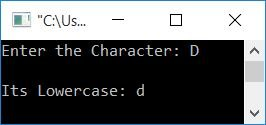Below is another sample run with user input d (already in lowercase):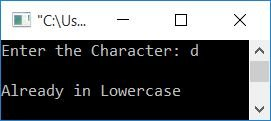And here is the final sample with user input 4 (not an alphabet):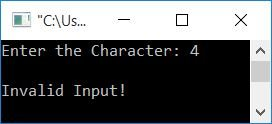## C++ Uppercase string to lowercase

Now let's convert the string from uppercase to lowercase. The following C++ program asks the user to enter a string, say "CODESCRACKER" (in uppercase), to change and print it into lowercase:

```#include<iostream>
#include<stdio.h>
using namespace std;
int main()
{
char strUpper="", strLower="";
int i=0, ascii, chk=0;
cout<<"Enter the String: ";
gets(strUpper);
while(strUpper[i]!='\0')
{
if(strUpper[i]>='A' && strUpper[i]<='Z')
{
ascii = strUpper[i];
ascii = ascii+32;
strLower[i] = ascii;
chk++;
}
else
strLower[i] = strUpper[i];
i++;
}
strLower[i]='\0';
if(chk==0)
else
cout<<"\nLowercase of String: "<<strLower;
cout<<endl;
return 0;
}```

Here's a sample run with string input: This is CodesCracker: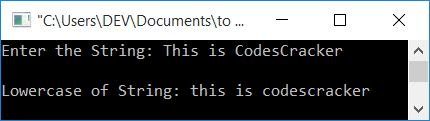## C++: Uppercase to Lowercase Using a Function

The question is: write a program in C++ that converts uppercase characters or strings to lowercase using the string function. The answer to this question is given below:

```#include<iostream>
#include<stdio.h>
#include<string.h>
using namespace std;
int main()
{
char str;
int len;
cout<<"Enter the Character/String: ";
gets(str);
len = strlen(str);
if(len==1)
cout<<"\nLowercase of Given Character: "<<strlwr(str);
else
cout<<"\nLowercase of Given String: "<<strlwr(str);
cout<<endl;
return 0;
}```

Here is its sample run with string input: CODESCRACKER: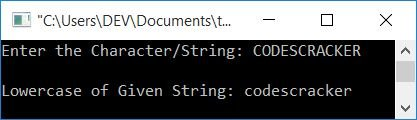#### The same program in different languages

C++ Quiz

« Previous Program Next Program »